# A photograph

A photograph will stick to a white square letter with a x cm length. The photo is 3/4 x cm long and 20 cm wide than the width of the paper. The surface of the remaining paper surrounding the photograph is 990 cm2. Find the size of paper and photo.

Correct result:

a =  39.846 cm
b =  29.885 cm
c =  20 cm

#### Solution:

$S_{1}=a^2 \ \\ b=3/4 \cdot \ a \ \\ c=20 \ \\ S_{2}=b \cdot \ c \ \\ S_{1}-S_{2}=990 \ \\ \ \\ a^2 - 3/4*a*20=990 \ \\ \ \\ a^2 - 3/4 \cdot \ a \cdot \ 20=990 \ \\ a^2 -15a -990=0 \ \\ \ \\ p=1; q=-15; r=-990 \ \\ D=q^2 - 4pr=15^2 - 4\cdot 1 \cdot (-990)=4185 \ \\ D>0 \ \\ \ \\ a_{1,2}=\dfrac{ -q \pm \sqrt{ D } }{ 2p }=\dfrac{ 15 \pm \sqrt{ 4185 } }{ 2 }=\dfrac{ 15 \pm 3 \sqrt{ 465 } }{ 2 } \ \\ a_{1,2}=7.5 \pm 32.345787979272 \ \\ a_{1}=39.845787979272 \ \\ a_{2}=-24.845787979272 \ \\ \ \\ \text{ Factored form of the equation: } \ \\ (a -39.845787979272) (a +24.845787979272)=0 \ \\ \ \\ a=a_{1}=39.8458=39.846 \ \text{cm}$

Checkout calculation with our calculator of quadratic equations.

$b=3/4 \cdot \ a=3/4 \cdot \ 39.8458=29.885 \ \text{cm}$
$c=20 \ \text{cm} \ \\ \ \\ S_{1}=a^2=39.8458^2 \doteq 1587.7037 \ \text{cm}^2 \ \\ S_{2}=b \cdot \ c=29.8845 \cdot \ 20=\dfrac{ 5977 }{ 10 }=597.7 \ \text{cm}^2$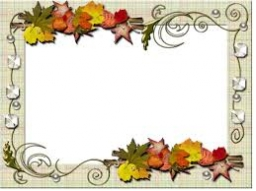Our examples were largely sent or created by pupils and students themselves. Therefore, we would be pleased if you could send us any errors you found, spelling mistakes, or rephasing the example. Thank you!

Please write to us with your comment on the math problem or ask something. Thank you for helping each other - students, teachers, parents, and problem authors.Tips to related online calculators
Looking for help with calculating roots of a quadratic equation?
Do you have a linear equation or system of equations and looking for its solution? Or do you have quadratic equation?

#### You need to know the following knowledge to solve this word math problem:

We encourage you to watch this tutorial video on this math problem:

## Next similar math problems:

• Four prismsQuestion No. 1: The prism has the dimensions a = 2.5 cm, b = 100 mm, c = 12 cm. What is its volume? a) 3000 cm2 b) 300 cm2 c) 3000 cm3 d) 300 cm3 Question No.2: The base of the prism is a rhombus with a side length of 30 cm and a height of 27 cm. The heig
• Square and circlesThe square in the picture has a side length of a = 20 cm. Circular arcs have centers at the vertices of the square. Calculate the areas of the colored unit. Express area using side a.
• Ratio of squaresA circle is given in which a square is inscribed. The smaller square is inscribed in a circular arc formed by the side of the square and the arc of the circle. What is the ratio of the areas of the large and small squares?
• Edge of prismThe regular quadrilateral prism has a surface of 250 dm2, its shell has a content of 200 dm2. Calculate its leading edge.What is the volume of a quadrilateral oblique prism with base edges of length a = 1m, b = 1.1m, c = 1.2m, d = 0.7m, if a side edge of length h = 3.9m has a deviation from the base of 20° 35 ´ and the edges a, b form an angle of 50.5°.
• The regularThe regular triangular prism has a base in the shape of an isosceles triangle with a base of 86 mm and 6.4 cm arms, the height of the prism is 24 cm. Calculate its volume.
• The funnel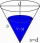The funnel has the shape of an equilateral cone. Calculate the content of the area wetted with water if you pour 3 liters of water into the funnel.
• Fountain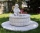The stone fountain, which has the shape of a cylinder with a diameter of 3 m, is 70 cm deep. How many m2 of stone is wetted with water?
• Cylinder containerThe cylindrical container with a diameter of 1.8 m contains 2,000 liters of water. How high does the water reach?
• Largest wallFind the content of the largest wall of a prism with the base of a rectangle which has a height of 4 dm, side c = 5 cm, and side b = 6 cm.
• Which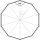Which of the following numbers most accurately area of a regular decagon with side s = 2 cm? (A) 9.51 cm2 (B) 20 cm2 (C) 30.78 cm2 (D) 31.84 cm2 (E) 32.90 cm2Calculate the content of a regular 15-sides polygon inscribed in a circle with radius r = 4. Express the result to two decimal places.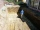Calculate how many cubic meters of soil needs to be removed from the excavation in the shape of an isosceles trapezoid, the top width is 3 meters, the lower width is 1.8 m, the depth of the excavation is 1 m, and the length is 20 m.One of the bases of the trapezoid is one-fifth larger than its height, the second base is 1 cm larger than its height. Find the dimensions of the trapezoid if its area is 115 cm2A regular quadrilateral pyramid has a volume of 24 dm3 and a base edge a = 4 dm. Calculate: a/height of the pyramid b/sidewall height c/surface of the pyramidFind the area of a shell of the regular hexagonal pyramid, if you know that its base edge is 5 cm long and the height of this pyramid is 10 cm.Find the volume of a regular hexagonal pyramid, the base edge of which is 12 cm long and the side edge 20 cm.